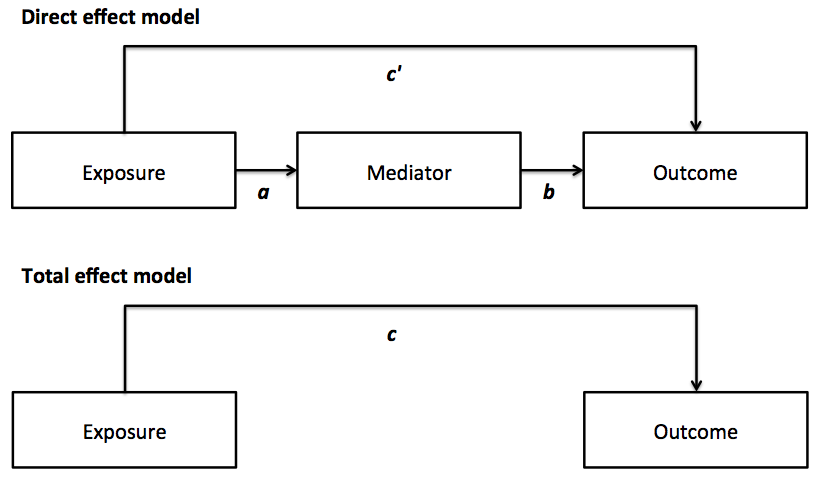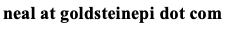# Neal D. Goldstein, PhD, MBI

May 31, 2016

### Epi Vignettes: Mediation frameworks and analysis

A brief synopsis of epidemiologic study design and methods with sample analytic code in R.

Almost hand in hand with moderation is the concept of mediation. Whereas moderation examines how a third variable influences the outcome based on an interaction with the exposure, mediation examines how a third variable "M" explains the relationship between the exposure "X" and outcome "Y". That is, the exposure leads to the intermediate variable (termed a mediator) that leads to the outcome, and may be useful to understand the how and why of the exposure effect. If the mediator fully explains this relationship, after including it in the analysis, the exposure would no longer independently be predictive of the outcome, as the mediator explained the full relationship. Assumed in this relationship is that the exposure has preceded the mediator, and the mediator preceded the outcome.

Just like moderation, mediation appears simple at first, but is like an onion with many layers dependent on your assumptions and parameterizations of variables. One of the seminal papers in the field of mediation analysis is the Baron and Kenny approach, outlined in "The Moderator-Mediator Variable Distinction in Social Psychological Research: Conceptual, Strategic, and Statistical Considerations" from 1986. In this paper the authors lay out a systems of equations (sometimes called the product approach) framework to modeling mediation through three separate and independent regression equations:

1. Outcome model without mediator (termed the total effect of X on Y): Y = X + covariates
2. Outcome model with mediator (termed the direct effect X on Y): Y = X + M + covariates
3. Mediator model: M = X + covariatesOne can then calculate the varying effects of the exposure and mediator in relation to the outcome, and compute a proportion mediated. The idea is if a mediator fully explains the relationship between an exposure and outcome, when "controlling" for the mediator in the total effect model, there will be no relationship between the exposure and outcome, as it is all explained by the mediating variable.

This approach has been widely used, due to its elegant simplicity. It is readily interpreted and understood by non-statisticians or epidemiologists. However, this approach also has several important limitations:

• The methods were built around linear regression models, therefore the outcome is necessarily parametric
• Precision estimates were limited to a single measure, e.g., using the Sobel method of calculating the standard error and statistical significance of the effects
• Assumes no (or uncontrolled) confounding between the exposure/outcome, exposure/mediator and mediator/outcome; further assumes that any mediator/outcome confounder is not affected by exposure
• Assumes no interaction between the mediator and exposure

Another framework has been offered for mediation analysis based on the counterfactual (or causal inference) approach. It posits the question: What would have happened to the outcome if the exposure (or mediator) were different for that individual? Of course this counterfactual condition cannot exist in nature, therefore we examine the average causal effects between those exposed and unexposed, assuming no other differences exist between groups. Users also need to be aware of the sequential ignorability assumption, which states that:

1. The assignment of exposure is statistically independent of the mediator and outcome. If the exposure was randomized this is theoretical upheld. If the exposure was naturally selected, as is the case in observation work, the proper potential confounders must be controlled for in the analysis.
2. The mediator is statistically independent of the exposure and outcome; in other words, the mediator should be viewed as a randomly distributed for the same exposure. As this is likely not the case, the proper covariates must be included as potential confounders. This assumption can potentially be tested through a sensitivity analysis.

This approach is attractive because it handles the aforementioned limitations, and rolls the mediation analysis into a single easy-to-use R command for both parametric and nonparametric models. In the presence of a linear outcome, the counterfactual approach should mirror the results from Barron and Kenny's systems of equation approach. As a potential drawback, the estimates from these methods may be less intuitive to interpret: they are on the predicted probability scale rather than the familiar log-odds scale when the outcome model is a generalized linear model.

There have also been many extensions to the product approach that either address its shortcomings or accommodate the counterfactual approach:

Regardless of the framework or statistical analysis, the analysis seeks to quantify mediation in terms of three effects:

1. Total effect: how the exposure affects the outcome overall
2. Direct effects: controlling for the mediation, how the exposure affects the outcome
3. Indirect (mediating) effects: how the mediator affects the outcome

The total effect is simply the sum of the direct and indirect effects. And based on this, one may calculate a proportion mediated, which is possibly the most intuitive metric from both of these approaches, as it quantifies how much of the exposure to outcome effect is driven by the mediator. A large amount suggests more mediation. For a recent guide on modern developments in mediation analysis, see this article by VanderWeele, although before diving into it, it helps to have some of the background as discussed in the more historic papers linked earlier in this post.

As an aside, occasionally the direct and indirect effects will compute with opposite signs (i.e., one will be positive, one will be negative), known as inconsistent mediation (also called suppression). In a classic example of the relationship between intelligence (X), boredom (M), and widget production (Y), the direct effect is positive as more intelligent workers may be more efficient at making widgets, but the mediating effect is negative, as more intelligent workers may become bored and make fewer widgets. In this instance, it is still possible to compute a proportion mediated, see footnote 3, by summing the absolute values of the direct and indirect effects, and dividing the absolute value of indirect effect by this sum: |Indirect| / (|Direct| + |Indirect|) * 100.

Sample codes in R

Systems of equations (Baron and Kenny approach) for dichotomous outcome

Outcome model without mediator

```eq1 = glm(Outcome ~ Exposure + Covariates, data=dataset, family=binomial(link="logit"))
c = as.numeric(coef(eq1)[" Exposure "])
```

Outcome model with mediator

```eq2 = glm(Outcome ~ Exposure + Mediator + Covariates, data=dataset, family=binomial(link="logit"))
c_prime = as.numeric(coef(eq2)["Exposure"])
b = as.numeric(coef(eq2)["Mediator"])
bSE = summary(eq2)\$coefficients["Mediator",2]
```

Mediator model for a dichotomous mediator

```eq3 = glm(Mediator ~ Exposure + Covariates, data=dataset, family=binomial(link="logit"))
a = as.numeric(coef(eq3)["Exposure"])
aSE = summary(eq3)\$coefficients["Exposure",2]
Summary statistics
summary(eq1)
summary(eq2)
summary(eq3)
```

Direct effect of a

```round(a,2)
round(exp(coef(eq3)),2)
round(exp(confint(eq3)),2)
```

Direct effect of b

```round(b,2)
round(exp(coef(eq2)),2)
round(exp(confint(eq2)),2)
```

Direct effect of c'

```round(c_prime,2)
round(exp(coef(eq2)),2)
round(exp(confint(eq2)),2)
```

Total effect of c

```round(c,2)
round(exp(coef(eq1)),2)
round(exp(confint(eq1)),2)
```

Percent mediated

```((c - c_prime) / c) * 100
```

Mediated/indirect effect

```c - c_prime #continuous
a*b #dichotomous, should approximate c - c_prime
round(a*b,2)
round(exp(a*b),2)
```

Sobel test for significance

```pooledSE = sqrt(((a^2)*(bSE^2))+((b^2)*(aSE^2)))
t = (a*b)/pooledSE
```

Check against normal distribution

```2*pnorm(-abs(t))
```

95% confidence

```exp((a*b) - (1.96*pooledSE))
exp((a*b) + (1.96*pooledSE))
```

Bootstrap for CI of indirect effect

```boot_ci = boot(dataset, bootIndirect, 1000, parallel="multicore", ncpus=4)
boot.ci(boot_ci, type="norm", index=1)

#bootstrap function, returns a*b from indirect model
bootIndirect = function(data,index)
{
bootdata = data[index,]
eq2 = glm(Outcome ~ Exposure + Mediator + Covariates, data=bootdata, family=binomial(link="logit"))
b = as.numeric(coef(eq2)["Mediator"])
eq3 = glm(Mediator ~ Exposure + Covariates, data=bootdata, family=binomial(link="logit"))
a = as.numeric(coef(eq3)["Exposure"])
return(a*b)
}
```

Counterfactual approach

Specify models

```model_outcome = glm(Outcome ~ Exposure + Mediator + Covariates, data=dataset, family=binomial(link="logit"))
model_mediator = glm(Mediator ~ Exposure + Covariates, family=binomial(link="logit"))
```

Run mediation analysis

```m.out = mediate(model_mediator, model_outcome, sims=1000, treat="Exposure", mediator="Mediator")
summary(m.out)
plot(m.out)
```

Values of interest

```#Total effect
#ACME (average) for indirect/mediated effect